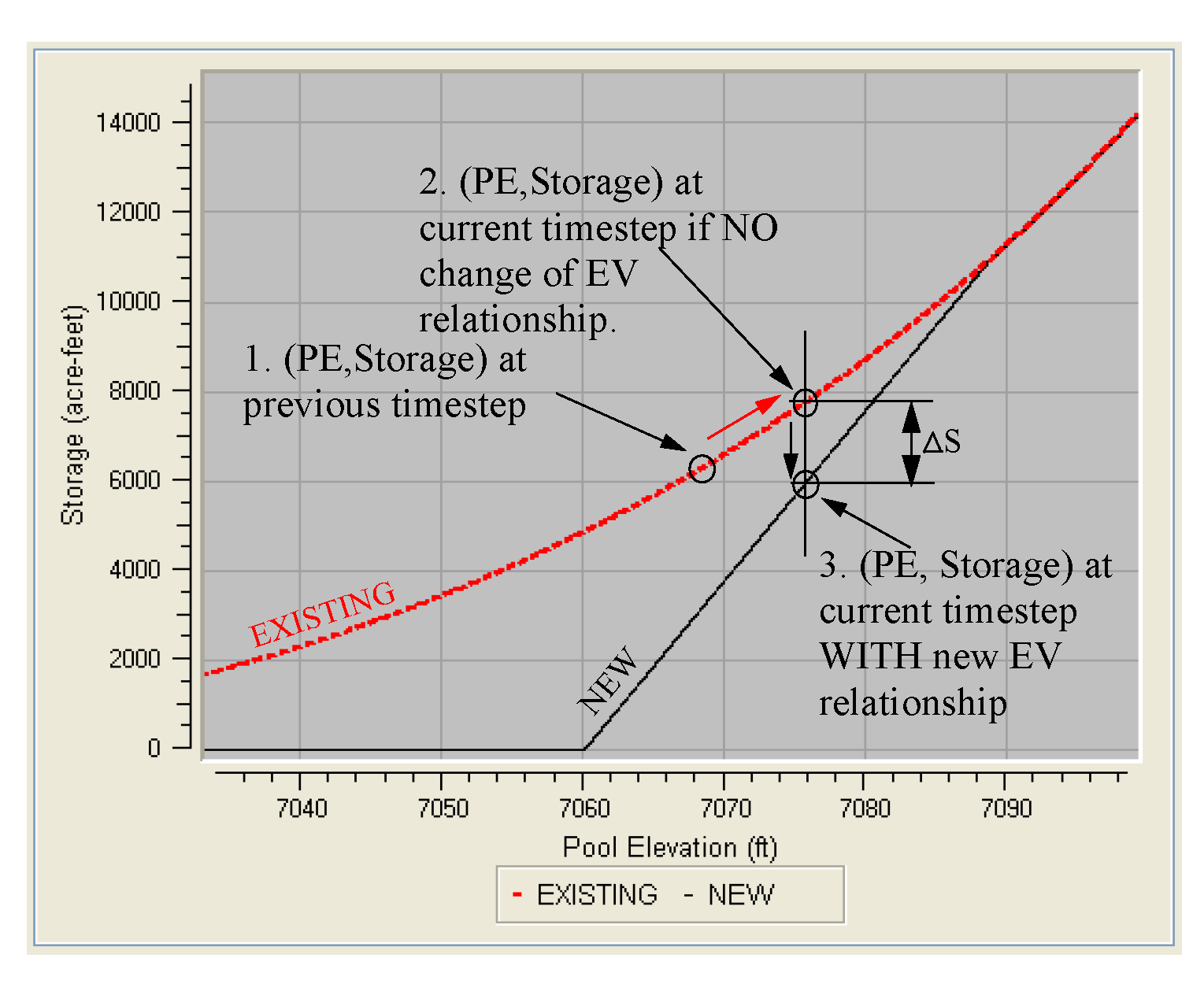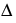Sediment
The Sediment category is used to enable algorithms which adjust reservoir Elevation Volume and possibly Elevation Area relationships in response to sediment inflow. See Time Varying Elevation Area for details on methods that change the elevation area relationship.None
The None method is the default for the Sediment category. No calculations are performed in this method.
There are no slots specific to this method.CRSS Sediment
The CRSS Sediment method is designed based on sedimentation calculations performed by the US Bureau of Reclamation’s Colorado River Simulation System (CRSS) model. This function distributes reservoir sediment based on the Empirical Area Reduction Method. Simply put, sediment is distributed through an iterative process in which a total volume loss due to sedimentation is calculated based on an assumed top of sediment elevation.
Slots Specific to This Method
Elevation Area Table
Type: Table
Units: LENGTH vs. AREA
Description: Generated elevation area table for calculating sediment distribution
Information:
I/O: Output only
Elevation Vol_Area Table Increment
Type: Table
Units: LENGTH
Description: Elevation increments for the generated Elevation Volume and Elevation Area Tables
Information: This table often needs more precise elevation increments than the sediment calculation tables.
I/O: Required input
Initial Elevation Area Table
Type: Table
Units: LENGTH vs. AREA
Description: Initial elevation area table
Information: Provided for comparison with initial data
I/O: Output only
Initial Elevation Volume Table
Type: Table
Units: LENGTH vs. VOLUME
Description: Initial elevation volume table
Information: provided for comparison with initial data
I/O: Output only
Sediment Distribution Coefficients
Type: Table
Units: NOUNITS
Description: Parameters for empirical equation governing sediment distribution
Information:
I/O: Required input
Sediment Inflow
Type: Series
Units: VOLUME
Description: Volume of sediment flowing into the reservoir at each timestep
Information:
I/O: Required input
User Input Elev Area Data
Type: Table
Units: LENGTH vs. AREA
Description: Initial Elevation Area relationship
Information: These values are initial conditions for the first timestep of the simulation. The elevation increments will be used for all sedimentation calculations.
I/O: Required input
Method Details
This volume loss is recalculated (with a new top of sediment elevation) at each iteration, until the calculated volume loss is equal to the actual volume of sediment inflow (within a specified convergence). The total volume loss calculation consists of a somewhat complicated algorithm utilizing elevation/area and elevation/volume data for the reservoir and an empirical equation. The empirical equation uses user specified parameters which relate the portion of total area that is taken up by sediment to the Pool Elevation. The empirical equation basically gives the shape of the accumulated sediment. The empirical equation has a close relationship to the elevation volume and elevation area characteristics of a given reservoir. The elevation/area and elevation/volume data is stored in a polynomial coefficient table, which gets recalculated after each timestep. The actual Elevation Area, Elevation Volume tables used by RiverWare are adjusted at the end of the sedimentation code (but prior to the hydrologic simulation).
Caution:  Take care when creating input data for this method. The close relationship between the empirical area reduction equation and the shape of the reservoir (reflected in the User Input Elev Area Data) makes the method fairly sensitive to input data. When choosing empirical parameters for this method, physical characteristics of the given reservoir need to be considered. The Bureau of Reclamation currently considers four possible types of reservoirs, with each type having a corresponding set of empirical area reduction parameters. The reservoir type classification is based on the shape of the Reservoir, the manner in which the reservoir is to be operated, and the size of the sediment particles to be deposited in the reservoir. The main emphasis is on the shape. Tables are used to classify the reservoirs based on these characteristics. Once the type has been established, the parameter values for that type can also be taken from tables in the literature. An incorrect set of parameters for a given reservoir will lead to an inability to achieve convergence on the sediment distribution within this method.Time Varying Elevation Volume
The Time Varying Elevation Volume method allows Elevation Volume (EV) relationships that change at specified times. The method is only available when the following default methods are selected in the following categories:
• Flood Control Release: only the None method is allowed
• Surcharge Release: only the None method is allowed
• Water Quality: None, Water Quality cannot be enabled
In Figure 25.2, both the existing and new EV relationships are plotted. Shown is a graphical example of how the mass balance should be performed on the timestep the EV relationship changes.
Figure 25.2The process is as follows.
1. This is the PE and Storage at the previous timestep. This is the starting condition.
2. On the current timestep, the Inflows and Outflows lead to a positive net inflow. This will increase the Pool Elevation. Using the existing table, a (PE, Storage) is calculated and shown as point 2.
3. The PE is used to interpolate a storage on the new EV table. The difference between storage at point 2 and point 3 is the loss of storage term. This means that the Pool Elevation is the same regardless of which table is used.
Slots Specific to This Method
Elevation Volume Table Time Varying
Type: Table Slot
Units: Length and volume
Description: Tables that represent the Elevation Volume relationship at various times in the run.
Information: The number of columns in this table should be set to one plus the number of times the Elevation Volume relationship changes. The column headings contain the date corresponding to the change. When you add a column to this slot, it is given a date later then the last column. You can set the date by selecting Column, then Set Column Value. You can then enter the date or use the date time selector to select the appropriate date. The dialog will only let you enter dates that correspond to timesteps. (Care should be exercised when switching model timesteps.) The column is then placed in the correct order compared to the other column labels. Each column should have an entry for each row in the Elevation column or an interpolation error may be issued during the run. The number of columns is equal to the number of changes plus one; that is, if there is one change then there should be two columns.
Following shows an example of a run that starts in 24:00 Jan 1, 1910 and the reservoir Elevation Volume changes three times. The column label of the first set of volumes must be on or before the initial timestep.
I/O: Input Only

Pool Elevation ft
24:00 Jan 1, 1910 Storage acre-ft
24:00 Jan 1, 1935 Storage acre-ft
24:00 Jan 1, 1953 Storage acre-ft
24:00 Jan 1, 1970 Storage acre-ft
5,100
0
0
0
0
5,120
10
9
8
7
5,150
15
14
13
12
5,200
20
19
18
17
5,250
25
24
23
22
Storage Adjustment from Elev Vol Table Change
Type: Series Slot
Units: VOLUME
Description: This is the volume of water that was lost to sedimentation
Information: The slot tracks the mass discontinuity that occurs when the Elevation Volume is changed because of a new reservoir Elevation Volume relationship. A positive number indicates storage was gained; a negative number indicates storage was lost.
I/O: Output only
Start of Run
The run proceeds as follows: at the start of the run, if the Time Varying Elevation Volume method is selected, a boolean variable isTimeVaryingElevVolume will be set to TRUE. At the same time, a pointer is set that specifies that all computations should use the Elevation Volume Table Time Varying. All computations involving the elevation volume relationship on the object use this pointer instead of the directly accessing the Elevation Volume Table. If the method is not selected, then the pointer is set to the Elevation Volume Table.
Note:  Even with this method selected, the original Elevation Volume Table is still visible. Although it is not used when dispatching or other simulation or rulebased simulation, it is still a general slot that is used in optimization calculations and water quality.
Start of Each Dispatch Method
At the start of each dispatch method, if the isTimeVaryingElevVolume is true, then the method will determine which column,“col”, of the table to use. It compares the current timestep to the column headings on the table and determine which column to use. For example, if the current timestep is March 3, 1940 for the table example above, then it will set col = 2 (column numbering is zero based) for use in all remaining computations. If the current timestep exactly matches one of the column headings, then an additional variable, isElevVolModDate, is set to TRUE and col is set to the that column minus 1. That is, for this dispatch, the previous relationship will be used, but will be adjusted at the end of the method.
Note:  If the Time Varying Elevation Volume method is not selected, then isTimeVaryingElevVolume and isElevVolModDate will remain false and the column to use on the Elevation Volume Table is set to 1. See Time Varying Elevation Volume for details.
The dispatch method then proceeds as normal using the computed “col” in all references to the specified elevation volume table.
End of Each Dispatch Method
At the end of the dispatch method (the description applies first to Solve given Inflow, Outflow), once Pool Elevation and Storage are known, if the isElevVolModDate is true, the method will lookup the Pool Elevation on the Elevation Volume Table Time Varying but this time use col+1 and get S’. The new storage S’ is the reduced storage using the new EV relationship. The difference,S = S - S’, and is set on the Storage Adjustment from Elev Vol Table Change slot. Then the Storage slot is set to S’ and PreviousStorage[t+1] is set to S’.
The preceding procedure describes the Solve given Inflow, Outflow. This same procedure is used for Solve given Inflow, Release. Once the new storage using the existing table is calculated, the new relationship can be used. If Pool Elevation is given (that is, Solve given Outflow, Pool Elevation), then the Pool Elevation is used to look up the storage using the existing and new tables. For the dispatch methods where Storage is known, the method will terminate the run with an error that the Time Varying Elevation Volume method cannot be used on a timestep where the storage is given.
Limitations
This method changes fundamental information about the reservoir. As a result, there are certain operations that cannot be used with this method including:
• Target operations that span table modification dates
• Dispatching the reservoir with any of the givenStorage methods on a modification date. Non modification dates can use the givenStorage methods.
• Any of the following RPL functions: StorageToElevation, ElevationToStorage, and StorageToArea. If these functions are called on a reservoir with the Time Varying Elevation Volume selected, an error message will be posted. Instead use the “...AtDate” version of that function. that is, use the StorageToElevationAtDate instead of the StorageToElevation function. Old models may need to be updated.
• There are many RPL functions like SolveOutflow, SolveStorage, GetMaxOutflowGivenInflow, etc that access the elevation volume relationship. These will access the correct table, but will always assume that the computation is being performed BEFORE any modifications to the relationship are made. That is, if you call the function and it is a modification timestep on the table, the function will use the previous column in all its computations. The relationship change is only considered at the end of the dispatch method, not in the RPL function.
Revised: 01/04/2021